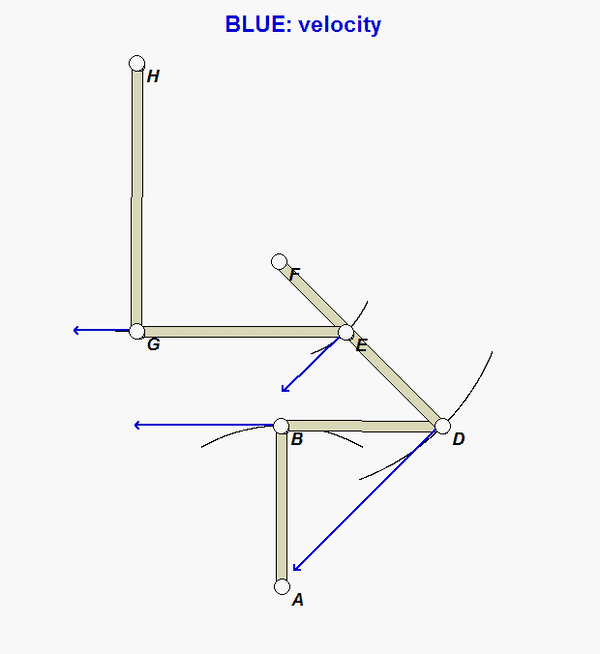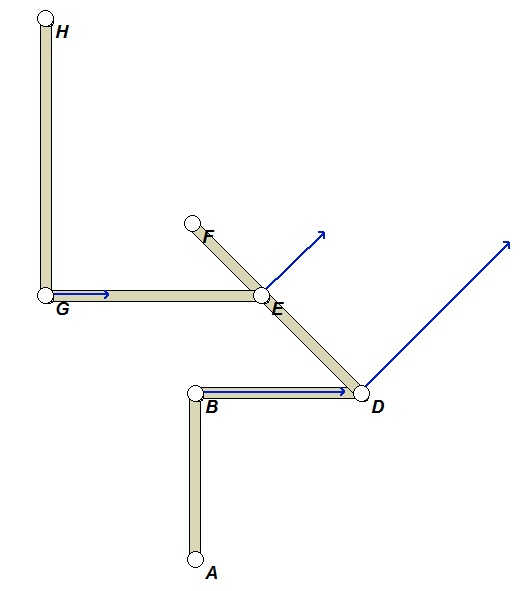# Homework H2.G - Sp23

 Problem statement Solution videoAsk and answer questions here through Comments. You can help others with thoughtful responses to their questions. And, you can learn from the process of doing so.

DISCUSSIONFor a general configuration of the mechanism:

• Note that points A, F and H are the instant centers (centers of rotation) for links AB, DF and GH.
• Because of this, the paths of B, D, E and G are circular arcs, as you can see in the animation below. The velocities of these points are tangent to their circular arc paths.
• Draw perpendiculars to the velocities of B and D. The intersection of these two perpendiculars defines the IC for link BD.
• Draw perpendiculars to the velocities of E and G. The intersection of these two perpendiculars defines the IC for link GE.
• The locations of these two ICs will guide you to the establishment of the relationships between the angular speeds of the links to the angular speed of input link, AB. It also guides you in determining the direction of rotation for each of the links.

For the configuration posed in this problem (shown below), where are the ICs for the links of the mechanism? Do you see the relationship between the location of these ICs and the directions of rotation for the links? Do you see the relationship between the location of these ICs and the speeds of the various pin joints in the mechanism (e.g., vD > vB and vE > vG)?Any questions??

## 35 thoughts on “Homework H2.G - Sp23”

1.Alicja Stoppel says:

For part c, are we supposed to leave it in terms of the distances from the point to the centers?

2.CMK says:

Use distances measured from the figure. You answers will depend on ratios of the lengths; therefore, you do not need to know the scale of the drawing, only that the figure was drawn proportionally.

3.Won Jun Lee says:

Do we need to measure the angle or can we assume the angles are 90 or 45 degrees?

1.CMK says:

The provided figure is drawn proportionally. Please measure distances, not angles. The goal is to not use trig functions, just distances from the figure.

1.John Reilly Kaufmann says:

So should I hold up a ruler to my computer screen and go from there? Or is there another way to measure the length of the arms, it seems unclear.

1.CMK says:

That would work, I suppose. Or, print out the problem statement and measure from there.

1.. says:

Should we also make a key explaining our values, or are there specific ones that we are supposed to get for an accurate answer to the problem?

4.Madeline B says:

I am having some trouble visualizing this problem. How do we know which path each link will take? Are we suppose to determine the perpendicular line based off the velocity of the motion for each link?

1.CMK says:

Consider first links AB, DF and GH. These links are pinned to ground at A, F and H, respectively. From this you know that the paths of B, D and G are semi-circular arcs centered at A, F and H, respectively.

You also know that the velocities of B, D and G are tangent to their respective paths. Start out with link BD: draw perpendiculars to the velocities of B and D. The IC for BD is at the intersection of these two perpendiculars.

Move on to DF. F travels on a semi-circular arc, with its velocity being tangent to that arc. Find the intersection of the perpendiculars to the velocities of of F and G - that intersection defines the IC for GE.

And so on...

5.Ryan McGuire Spees says:

For part C, how can we find the omega ratio when velocity is unknown to us? I understand how we can approximate the distance from the instant centers, but the velocities are not solvable for the equation Va = Ra/o * omega

1.CMK says:

All of the velocities are related to angular velocities through the ICs. In the end, you will have ratios of only the omegas.

6.Michael Bradley Mayhew says:

Since the points A, F, and H are fixed, we should only have ICs for GE and BD correct? The velocities being zero at the fixed points would mean there isn't a perpendicular line to form an IC.

1.CMK says:

Note that A, F and H are the ICs for links AB, FD and HG.

7.William Trent Allen says:

Will there be one instant center for the entire mechanism?

1.Qianyu Zhao says:

As far as I know, not likely. Since it would mean all components rotate about that instant center at certain moment. For example, if we only look at link AB, BD and DF in this problem, which make it a simple 3-bar linkage. At this moment, although BD and DF share the same instant center, AB doesn’t. As a result, it won’t allow us to have an instant center for every single link and thus there’s no instant center for the whole structure.

1.Qianyu Zhao says:

A 3-bar closed loop might work, but that will be rigid.

2.Drew Thomas Casterton says:

You should get an instant center for each bar, not the whole system. Remembering that constrained bars have a velocity vector normal to the bar at the end opposite they are constrained should be helpful.

8.Carter Casbon says:

I understand why vD > vB, vE > vG, and vD > vE, but how can the relationship between vB and vG be established?

1.CMK says:

These are all established by distances of the points from the ICs on the respective links.

2.Melissa Jewel Brock says:

How can you tell that vB < vD, vG<vE, and vE<vD?

1.CMK says:

Melissa:

Look at the location of the IC for link BD. Since D is further from this IC than is B, then vD > vB.

Look at the location of the IC for link EG. Since E is further from this IC than is G, then vE > vG.

Look at the location of the IC for link DF. Since D is further from this IC than is E, then vD > vE.

Does that make sense?

9.J Cena says:

If my monitor has the pdf open with scale set to 100% are those the accurate distances, and can I put a ruler up to my monitor and get the distances?

1.Drew Thomas Casterton says:

Yes, that should work. I know it seems weird at first, but you should find that the scale between the two bars which are asked about is a value you would expect to find in a well written problem.

10.Allie says:

I understand how to get v_B from AB, v_D from BD, and v_G from GH, but I am at a loss for how to get v_E, as well as how you could possibly equate these values if you had them all.

How do you find v_E and how do you equate the values to find the ratio? I have the approximate lengths to all of the links, but I am still at a loss.

1.CMK says:

Allie: Please keep in mind that E is on link FD, with FD rotating about F (i.e., F is the IC for FD). The speed of E, vE, is the distance FE x angular speed of FD.

1.Clairice Holmes says:

Why would v_E = the distance FD x angular speed of FD? I am confused because I thought v_E would be equal to omega_FD*r_E/F? Since F is the instant center for link FD, and EF is the distance from the velocity of the point we want to the instant center.

1.CMK says:

Sorry. I mistyped.

11.Christian Ladehoff says:

To determine a sense of rotation (CW or CCW), do we just look at the animation and velocity direction and go from there or is there a mathematical way to get the direction?

1.CMK says:

Look at two things: the location of the IC and the direction of velocity for one point on that link. For example, the IC for BD is at F and we know that B is moving to the right. Since BD is rotating about F and B is moving to the right, the BD is rotating CCW.

Yes, you can use the animation to check your answers; however, reason out your answers first without looking at the animation. You will not have the animations on your exam.

12.Justin James Paluch says:

I am really confused about how to find the ratio of the angular velocities for part C. Any guidance/direction would be greatly appreciated. Thanks!

1.Henry Kamis says:

I would start by finding Vb = omega AB * R_ AB, and set that equal to omega BD * R_BF. After solving for omega BD in terms of AB, repeat this process by finding Vd, Ve, and Vg. At the end you will find Omega GH in terms of all the distances (R_AB, R_BF, etc.) and divide off the Omega AB. This will provide you with a numerical answer. Hope this helped!

13.Jacob says:

When finding the ratio in part C are we supposed to account for the opposite directions of w_GH and w_AB?

1.CMK says:

The omegas listed in the problem statements are speeds, numbers without signs.

14.Allie says:

I am almost done with this problem. I found the relationships relating omega_AB, omega_BD, and omaga_DF. I also found the relationship between omega_GH and omega_GE, but I can't find how to connect the relationships (like how to relate omega_DF and omega_GE). How can I do this? The only way I can think of is writing the equation for v_E on link EG, but this uses r_E/E, which is equal to 0, so I don't think this makes sense.

1.Henry Kamis says:

The way I did it was to relate all the velocities and omega values. To relate omega DF to omega EG specifically, I solved for Ve = omega DF * R_EF = omega EG * R_EJ. (J was what I called the point just below H). After doing this process a few more times in terms of omega AB, you'll divide by omega AB and cancel it out to find a number.